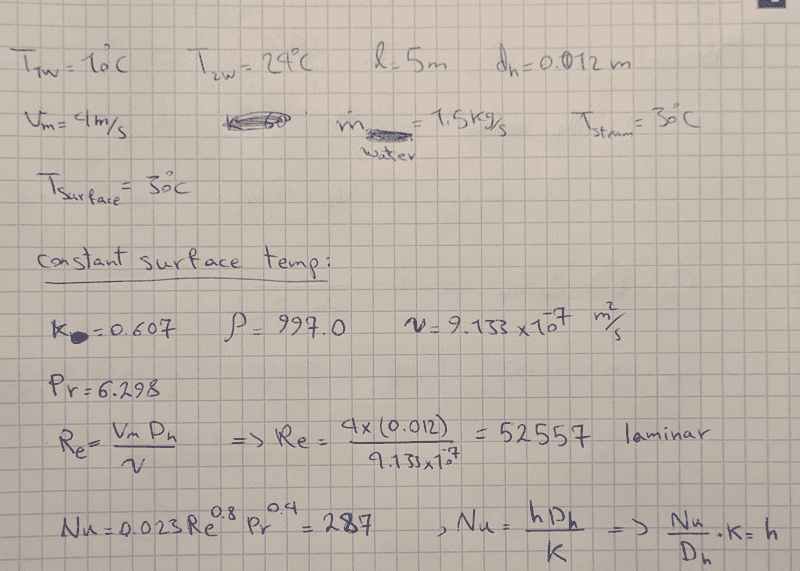# Average heat transfer coefficient (forced convection)

bardia sepehrnia
Homework Statement:
Cooling water available at 10C is used to condense steam at 30C in the condenser of a power plant at a rate of 0.15kg/s by circulating the cooling water through a bank of 5-m-long 1.2cm-internal-diameter thin copper tubes. Water enters the tubes at a mean velocity of 4m/s, and leaves at a temperature of 24C. The tubes are nearly isothermal at 30 C. Determine the average heat transfer coefficient between the water and the tubes and the number of tubes needed to achieve the indicated heat transfer rate in the condenser.
Relevant Equations:
Q=mcdeltaT, , Q=h*As*deltaTln, Re=(Vmean*Dh)/kinematic viscocity
Nu=0.023(Re^0.8)*(Pr^0.4), Nu=(h*Dh)/k
So firstly, I don't understand if the mass flow rate is for steam or for water. If it is for water, I know I can find the heat transfer rate using equation:Q=mcdeltaT.
But then I don't know how to find h (the average heat transfer coefficient) because I don't know the surface area (As). I can find the log mean temperature difference but there are still 2 unknowns in the equation: Q=h*As*deltaTln. Surface area and average heat transfer coefficient.

I also can calculate the Reynold number and ultimately calculate the Nusselt's number, but I still can't find out h because h=(Nu*Dh/k) which means h is function of diameter, and the diameter of 1 tube is known but since we don't know how many tubes is required, then the Dh is also unknown.

Any help will be appreciated.

My attempt with the solution and trying to use Nusselt number to find h.Homework Helper
Hi,
if the mass flow rate is for steam or for water
If it's for water, you know the number of pipes from the velocity, isn't it ?

And if it's for steam, you have ##Q## and you also have the number of pipes.

Could it be the exercise is a lot easier than you think ? I remember something like $${1\over U} = {1\over h_{\text{w}} + {1\over \lambda} + {1\over h_{\text{st}}$$and the 'The tubes are nearly isothermal at 30 C' means the last two terms are negligible ?

@Chestermiller, am I making sense ?

##\ ##

Mentor
You know the Nussult number and you know the tube diameter, so you know the heat transfer coefficient. Simple as that. The hydraulic diameter is equal to the tube diameter no matter how many tubes you have.

Incidentally, you indicate that for a Re of 50000, the flow is laminar. That is not correct. It is turbulent.

Last edited:
•BvU
bardia sepehrnia
You know the Nussult number and you know the tube diameter, so you know the heat transfer coefficient. Simple as that. The hydraulic diameter is equal to the tube diameter no matter how many tubes you have.

Incidentally, you indicate that for a Re of 50000, the flow is laminar. That is not correct. It is turbulent.
The mass flow rate is for steam, but I still don't understand how to solve the question. You say that the hydraulic diameter is equal to tube diameter no matter how many tubes I have. Obviously the hydraulic diameter is always the same, but h (convection heat transfer coefficient), is a function of hydraulic diameter. So if we have 10 tubes, or 1 tube, the heat transfer coefficient should change. You can't have same heat transfer coefficient for 1 tube and 10 tubes. Makes no sense.

bardia sepehrnia
I asked my professor and he said it's for the steam, but I still don't know how to solve this question and what you said didn't help at all.

Mentor
The mass flow rate is for steam, but I still don't understand how to solve the question. You say that the hydraulic diameter is equal to tube diameter no matter how many tubes I have. Obviously the hydraulic diameter is always the same, but h (convection heat transfer coefficient), is a function of hydraulic diameter. So if we have 10 tubes, or 1 tube, the heat transfer coefficient should change. You can't have same heat transfer coefficient for 1 tube and 10 tubes. Makes no sense.
Wrong! The hydraulic diameter is a geometric parameter equal to 4 times volume of tubes divided by the wetted heat transfer area: $$D_h=\frac{4\left(\frac{\pi D^2}{4}L\right)n}{(\pi D L)n}=D$$So, $$h=\frac{(287)k}{D}$$

1. Based on the steam condensing in the condenser, what is the heat load of the condenser?

2. What water flow rate do you need to match this heat load with the water entering at 10 C and exiting at 24 C?

3. Based on the tube cross sectional area and water velocity, what is the water flow rate per tube?

4. How many tubes are there?

Last edited:
Mentor
I asked my professor and he said it's for the steam, but I still don't know how to solve this question and what you said didn't help at all.
Please don't insult me by implying that I don't know how to solve heat transfer problems, even a simple one like this. When I said that the hydraulic diameter is equal, in this case, to the tube diameter, I wasn't guessing. Now if that didn't help at all, then I don't know what will!